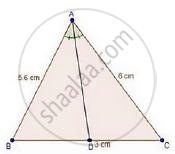Share

In a δAbc, Ad is the Bisector of ∠A, Meeting Side Bc at D. If Ab = 5.6 Cm, Ac = 6cm and Dc = 3cm, Find Bc. - CBSE Class 10 - Mathematics

Question

In a ΔABC, AD is the bisector of ∠A, meeting side BC at D.

If AB = 5.6 cm, AC = 6cm and DC = 3cm, find BC.

SolutionWe have, In ΔABC, AD is the bisector of ∠A.

We know that, the internal bisector of an angle of a triangle divides the opposite side internally in the ratio of the sides containing the angle.

therefore"BD"/"DC"="AB"/"AC"

rArr"BD"/3=5.6/6

rArr"BD"=(5.6xx3)/6=5.6/2=2.8  cm

⇒ BD = 2.8 cm

Since, BC = BD + DC

= 2.8 + 3

= 5.8 cm

∴ BC = 5.8 cm

Is there an error in this question or solution?

Video TutorialsVIEW ALL 

Solution In a δAbc, Ad is the Bisector of ∠A, Meeting Side Bc at D. If Ab = 5.6 Cm, Ac = 6cm and Dc = 3cm, Find Bc. Concept: Angle Bisector.
S